# 13.ppt

16 May 2023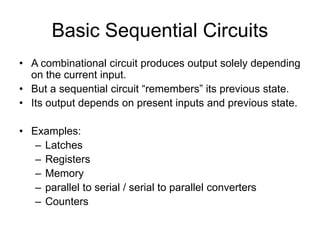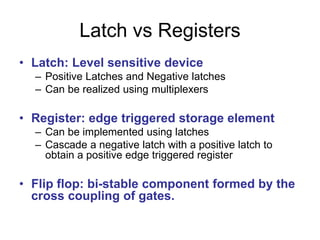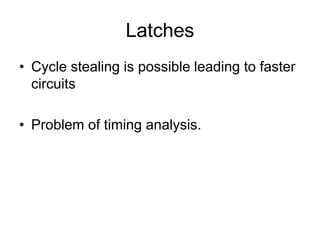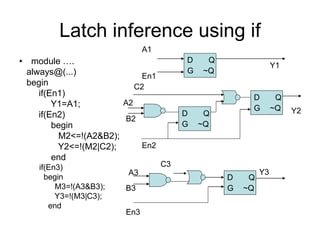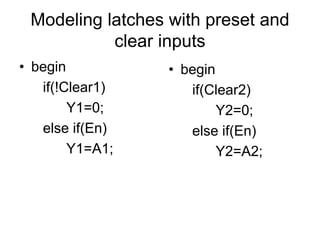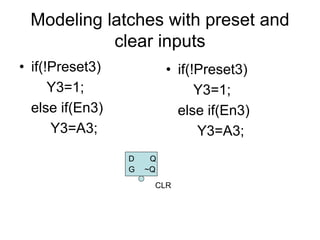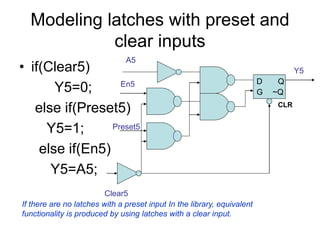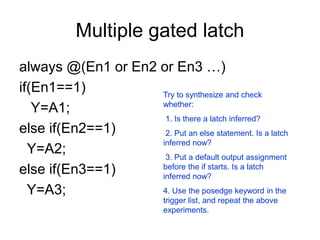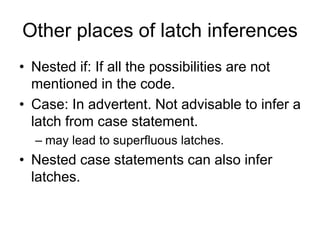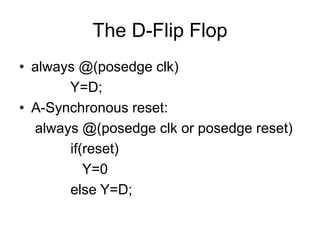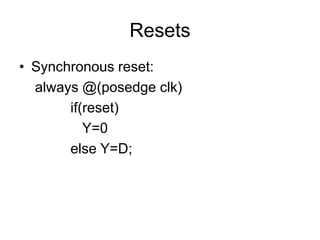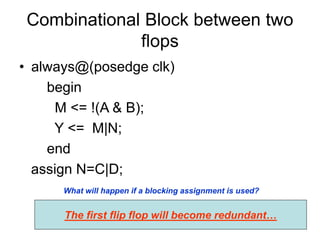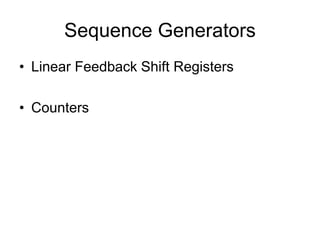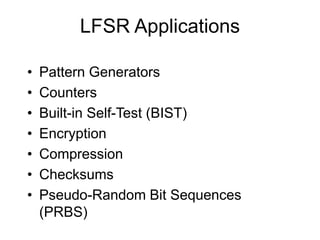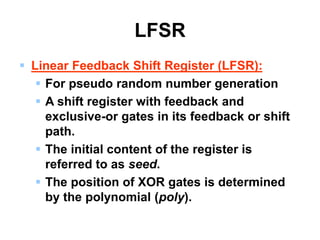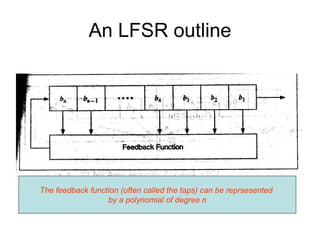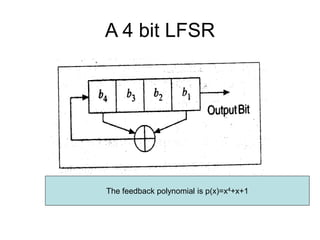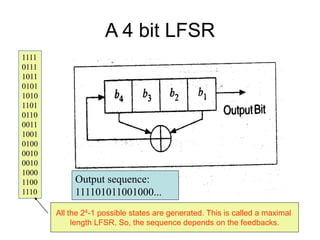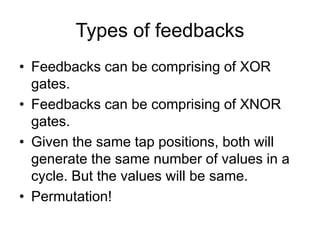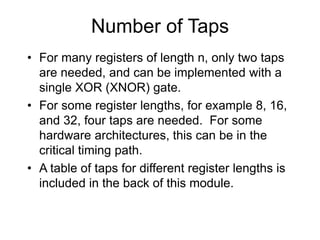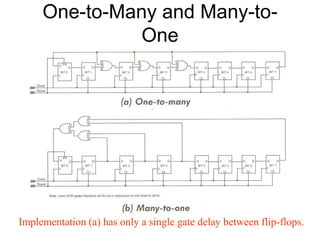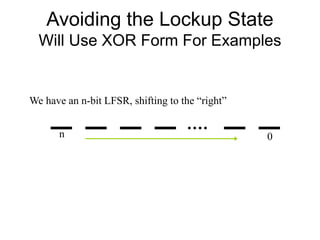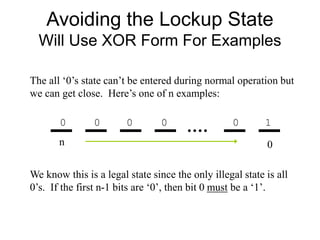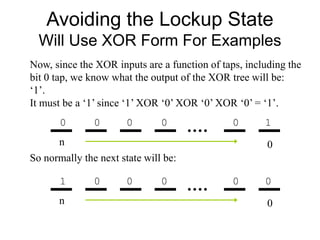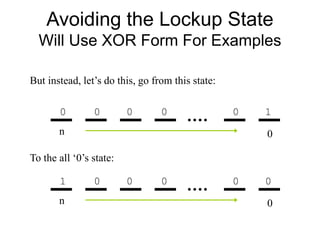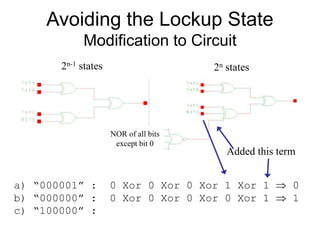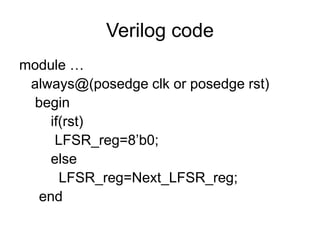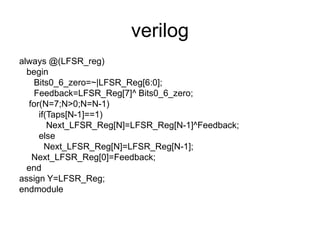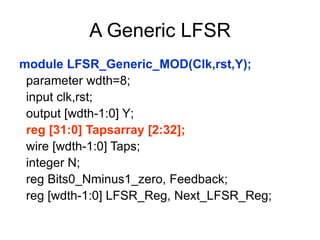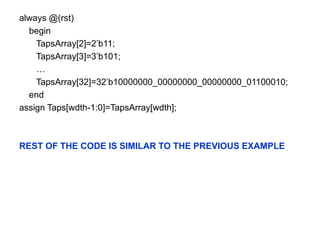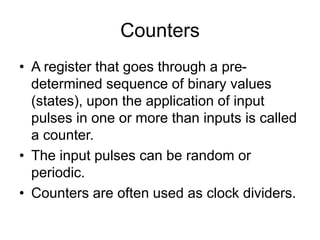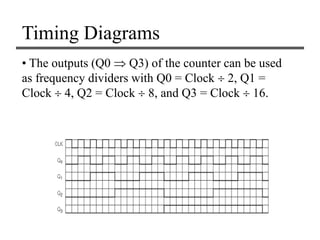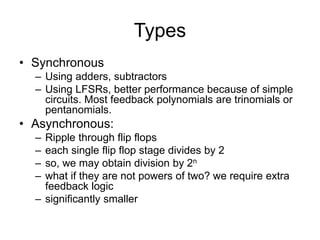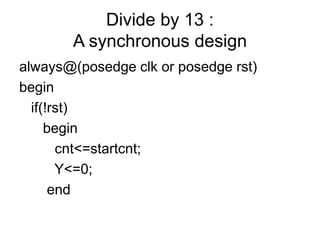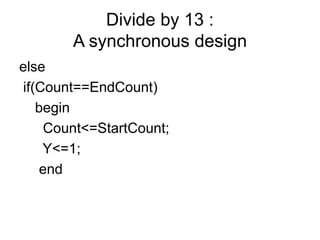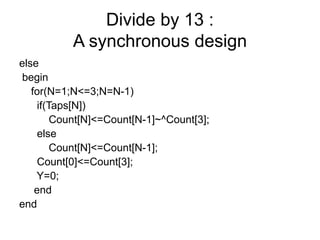1 sur 37

### 13.ppt

• 1. 1 Modeling Synchronous Logic Circuits Debdeep Mukhopadhyay Associate Professor Dept of Computer Science and Engineering NYU Shanghai and IIT Kharagpur
• 2. Basic Sequential Circuits • A combinational circuit produces output solely depending on the current input. • But a sequential circuit “remembers” its previous state. • Its output depends on present inputs and previous state. • Examples: – Latches – Registers – Memory – parallel to serial / serial to parallel converters – Counters
• 3. Latch vs Registers • Latch: Level sensitive device – Positive Latches and Negative latches – Can be realized using multiplexers • Register: edge triggered storage element – Can be implemented using latches – Cascade a negative latch with a positive latch to obtain a positive edge triggered register • Flip flop: bi-stable component formed by the cross coupling of gates.
• 4. Latches • Cycle stealing is possible leading to faster circuits • Problem of timing analysis.
• 5. Latch inference using if • module …. always@(...) begin if(En1) Y1=A1; if(En2) begin M2<=!(A2&B2); Y2<=!(M2|C2); end if(En3) begin M3=!(A3&B3); Y3=!(M3|C3); end D G Q ~Q A1 En1 Y1 D G Q ~Q D G Q ~Q A2 B2 C2 En2 Y2 A3 B3 C3 D G Q ~Q En3 Y3
• 6. Modeling latches with preset and clear inputs • begin if(!Clear1) Y1=0; else if(En) Y1=A1; • begin if(Clear2) Y2=0; else if(En) Y2=A2;
• 7. Modeling latches with preset and clear inputs • if(!Preset3) Y3=1; else if(En3) Y3=A3; • if(!Preset3) Y3=1; else if(En3) Y3=A3; D G Q ~Q CLR
• 8. Modeling latches with preset and clear inputs • if(Clear5) Y5=0; else if(Preset5) Y5=1; else if(En5) Y5=A5; D G Q ~Q Clear5 Preset5 En5 A5 Y5 If there are no latches with a preset input In the library, equivalent functionality is produced by using latches with a clear input. CLR
• 9. Multiple gated latch always @(En1 or En2 or En3 …) if(En1==1) Y=A1; else if(En2==1) Y=A2; else if(En3==1) Y=A3; Try to synthesize and check whether: 1. Is there a latch inferred? 2. Put an else statement. Is a latch inferred now? 3. Put a default output assignment before the if starts. Is a latch inferred now? 4. Use the posedge keyword in the trigger list, and repeat the above experiments.
• 10. Other places of latch inferences • Nested if: If all the possibilities are not mentioned in the code. • Case: In advertent. Not advisable to infer a latch from case statement. – may lead to superfluous latches. • Nested case statements can also infer latches.
• 11. The D-Flip Flop • always @(posedge clk) Y=D; • A-Synchronous reset: always @(posedge clk or posedge reset) if(reset) Y=0 else Y=D;
• 12. Resets • Synchronous reset: always @(posedge clk) if(reset) Y=0 else Y=D;
• 13. Combinational Block between two flops • always@(posedge clk) begin M <= !(A & B); Y <= M|N; end assign N=C|D; What will happen if a blocking assignment is used? The first flip flop will become redundant…
• 14. Sequence Generators • Linear Feedback Shift Registers • Counters
• 15. LFSR Applications • Pattern Generators • Counters • Built-in Self-Test (BIST) • Encryption • Compression • Checksums • Pseudo-Random Bit Sequences (PRBS)
• 16. LFSR  Linear Feedback Shift Register (LFSR):  For pseudo random number generation  A shift register with feedback and exclusive-or gates in its feedback or shift path.  The initial content of the register is referred to as seed.  The position of XOR gates is determined by the polynomial (poly).
• 17. An LFSR outline The feedback function (often called the taps) can be reprsesented by a polynomial of degree n
• 18. A 4 bit LFSR The feedback polynomial is p(x)=x4+x+1
• 19. A 4 bit LFSR 1111 0111 1011 0101 1010 1101 0110 0011 1001 0100 0010 0010 1000 1100 1110 Output sequence: 111101011001000... All the 24-1 possible states are generated. This is called a maximal length LFSR. So, the sequence depends on the feedbacks.
• 20. Types of feedbacks • Feedbacks can be comprising of XOR gates. • Feedbacks can be comprising of XNOR gates. • Given the same tap positions, both will generate the same number of values in a cycle. But the values will be same. • Permutation!
• 21. Number of Taps • For many registers of length n, only two taps are needed, and can be implemented with a single XOR (XNOR) gate. • For some register lengths, for example 8, 16, and 32, four taps are needed. For some hardware architectures, this can be in the critical timing path. • A table of taps for different register lengths is included in the back of this module.
• 22. One-to-Many and Many-to- One Implementation (a) has only a single gate delay between flip-flops.
• 23. Avoiding the Lockup State Will Use XOR Form For Examples We have an n-bit LFSR, shifting to the “right” 0 n
• 24. Avoiding the Lockup State Will Use XOR Form For Examples The all ‘0’s state can’t be entered during normal operation but we can get close. Here’s one of n examples: 0 n 0 0 0 0 0 1 We know this is a legal state since the only illegal state is all 0’s. If the first n-1 bits are ‘0’, then bit 0 must be a ‘1’.
• 25. Avoiding the Lockup State Will Use XOR Form For Examples Now, since the XOR inputs are a function of taps, including the bit 0 tap, we know what the output of the XOR tree will be: ‘1’. It must be a ‘1’ since ‘1’ XOR ‘0’ XOR ‘0’ XOR ‘0’ = ‘1’. 0 n 0 0 0 0 0 1 So normally the next state will be: 0 n 1 0 0 0 0 0
• 26. Avoiding the Lockup State Will Use XOR Form For Examples But instead, let’s do this, go from this state: 0 n 0 0 0 0 0 1 To the all ‘0’s state: 0 n 1 0 0 0 0 0
• 27. Avoiding the Lockup State Modification to Circuit NOR of all bits except bit 0 2n-1 states 2n states Added this term a) “000001” : 0 Xor 0 Xor 0 Xor 1 Xor 1  0 b) “000000” : 0 Xor 0 Xor 0 Xor 0 Xor 1  1 c) “100000” :
• 28. Verilog code module … always@(posedge clk or posedge rst) begin if(rst) LFSR_reg=8’b0; else LFSR_reg=Next_LFSR_reg; end
• 30. A Generic LFSR module LFSR_Generic_MOD(Clk,rst,Y); parameter wdth=8; input clk,rst; output [wdth-1:0] Y; reg [31:0] Tapsarray [2:32]; wire [wdth-1:0] Taps; integer N; reg Bits0_Nminus1_zero, Feedback; reg [wdth-1:0] LFSR_Reg, Next_LFSR_Reg;
• 32. Counters • A register that goes through a pre- determined sequence of binary values (states), upon the application of input pulses in one or more than inputs is called a counter. • The input pulses can be random or periodic. • Counters are often used as clock dividers.
• 33. Timing Diagrams • The outputs (Q0  Q3) of the counter can be used as frequency dividers with Q0 = Clock  2, Q1 = Clock  4, Q2 = Clock  8, and Q3 = Clock  16.
• 34. Types • Synchronous – Using adders, subtractors – Using LFSRs, better performance because of simple circuits. Most feedback polynomials are trinomials or pentanomials. • Asynchronous: – Ripple through flip flops – each single flip flop stage divides by 2 – so, we may obtain division by 2n – what if they are not powers of two? we require extra feedback logic – significantly smaller
• 35. Divide by 13 : A synchronous design always@(posedge clk or posedge rst) begin if(!rst) begin cnt<=startcnt; Y<=0; end
• 36. Divide by 13 : A synchronous design else if(Count==EndCount) begin Count<=StartCount; Y<=1; end
• 37. Divide by 13 : A synchronous design else begin for(N=1;N<=3;N=N-1) if(Taps[N]) Count[N]<=Count[N-1]~^Count; else Count[N]<=Count[N-1]; Count<=Count; Y=0; end end